Ex 7.3

Chapter 7 Class 11 Permutations and Combinations (Term 2)
Serial order wise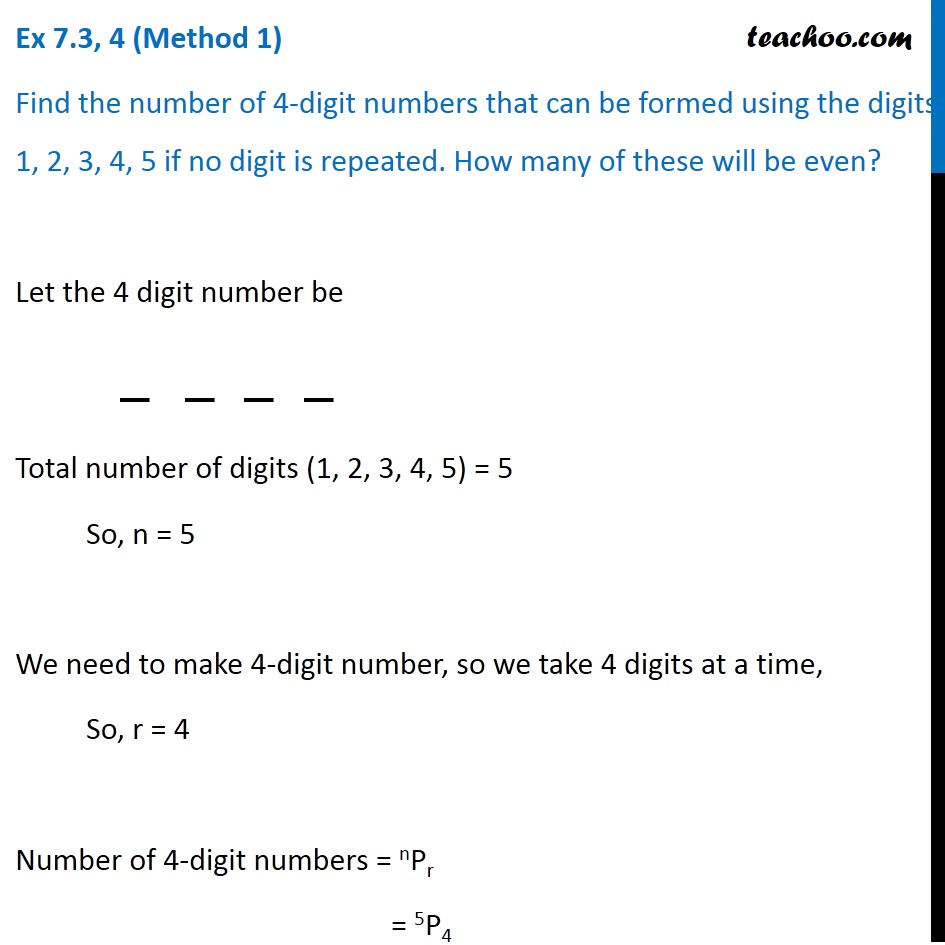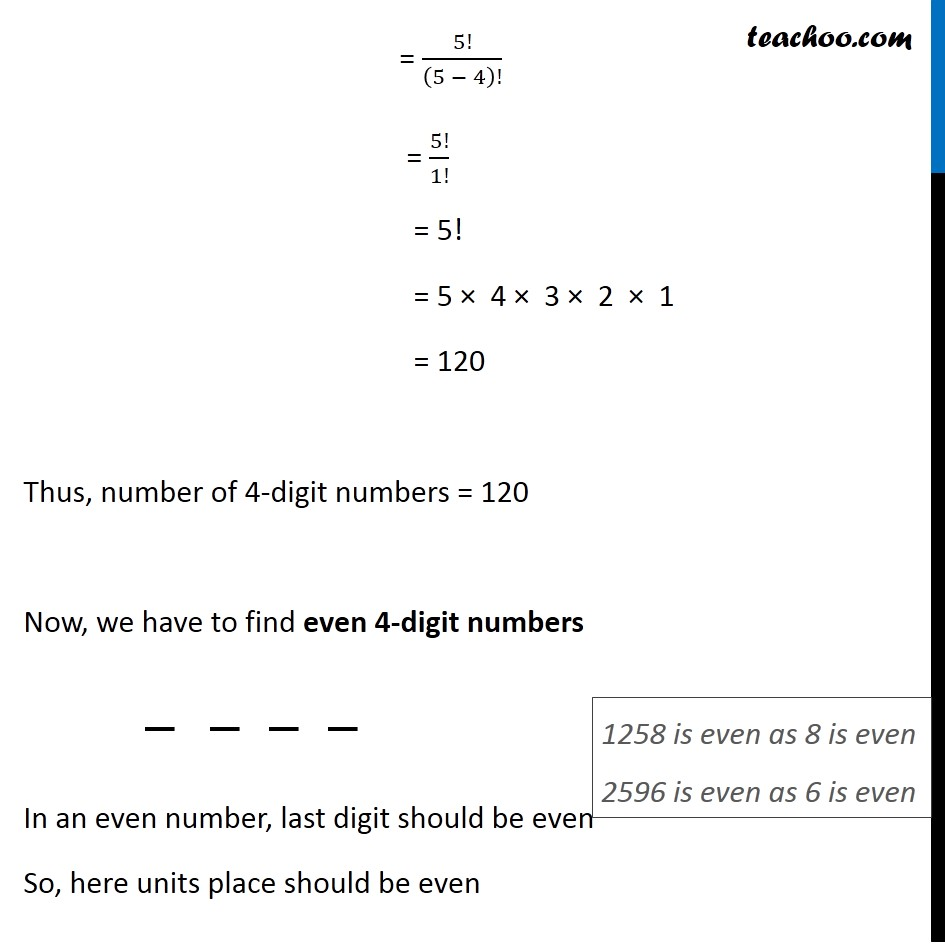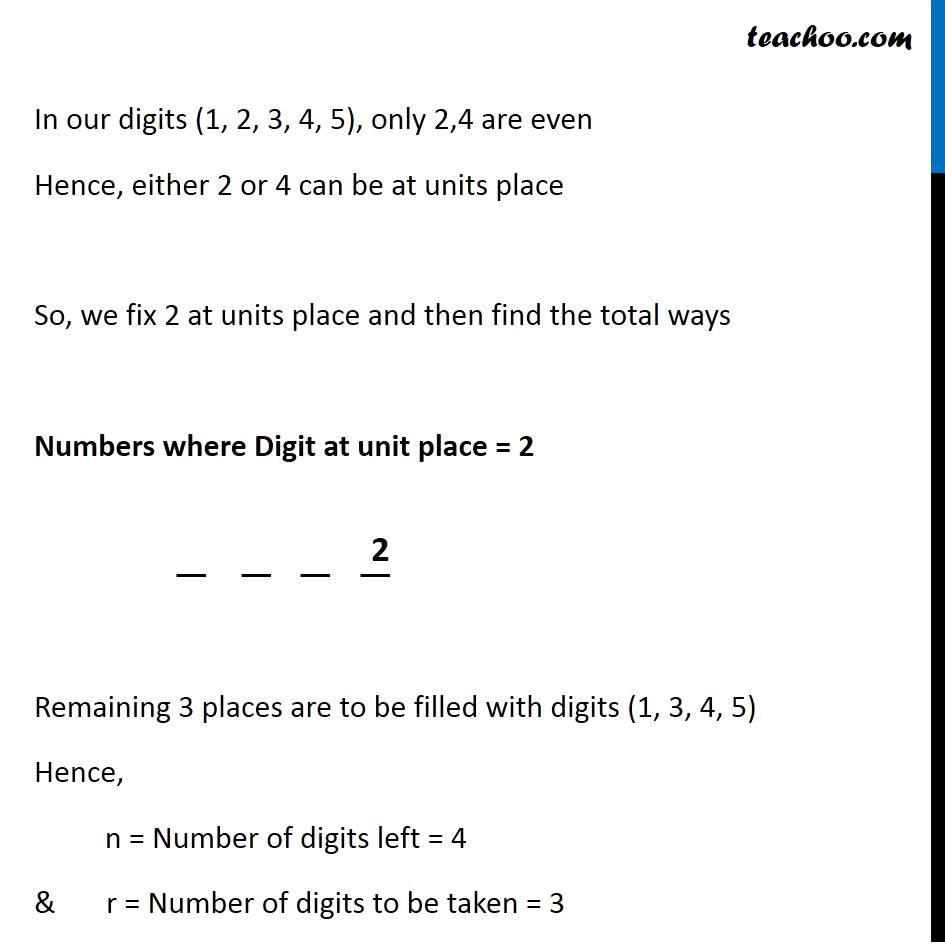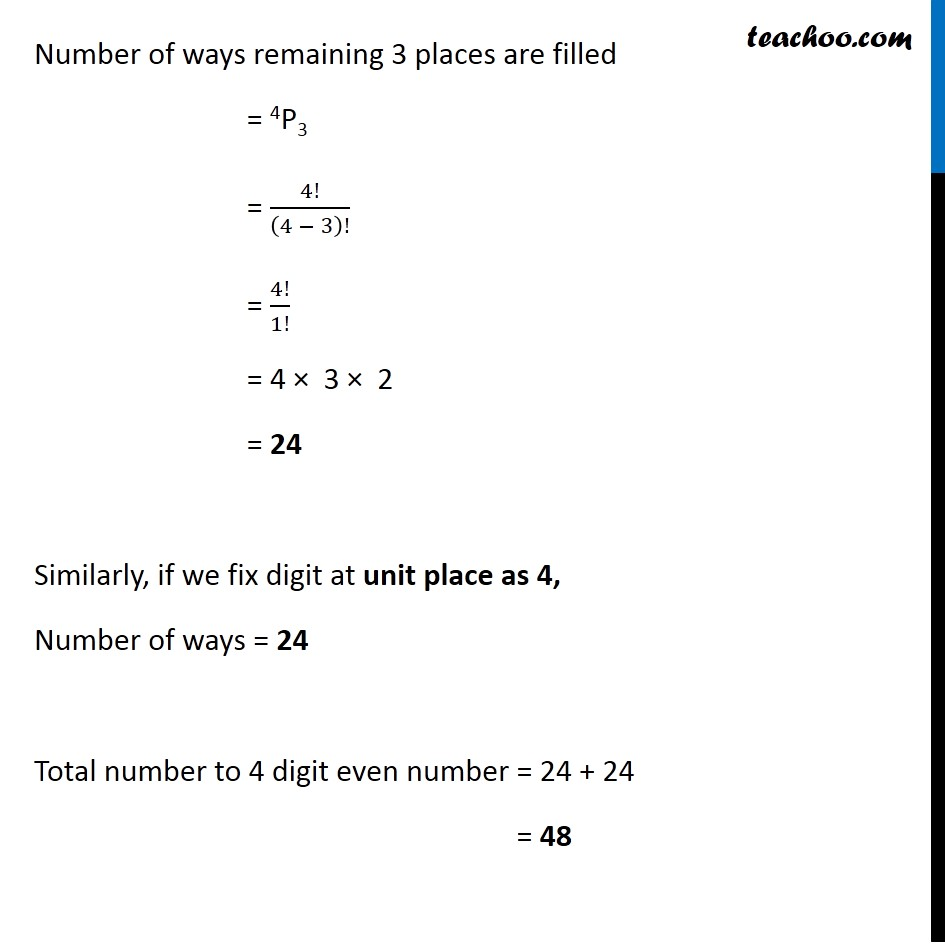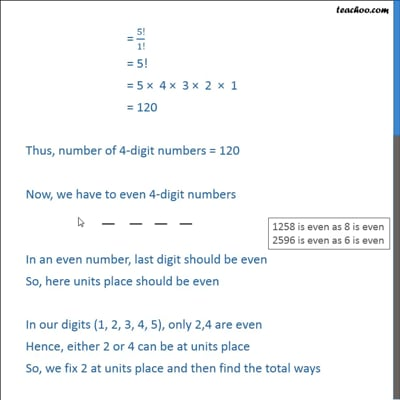This video is only available for Teachoo black users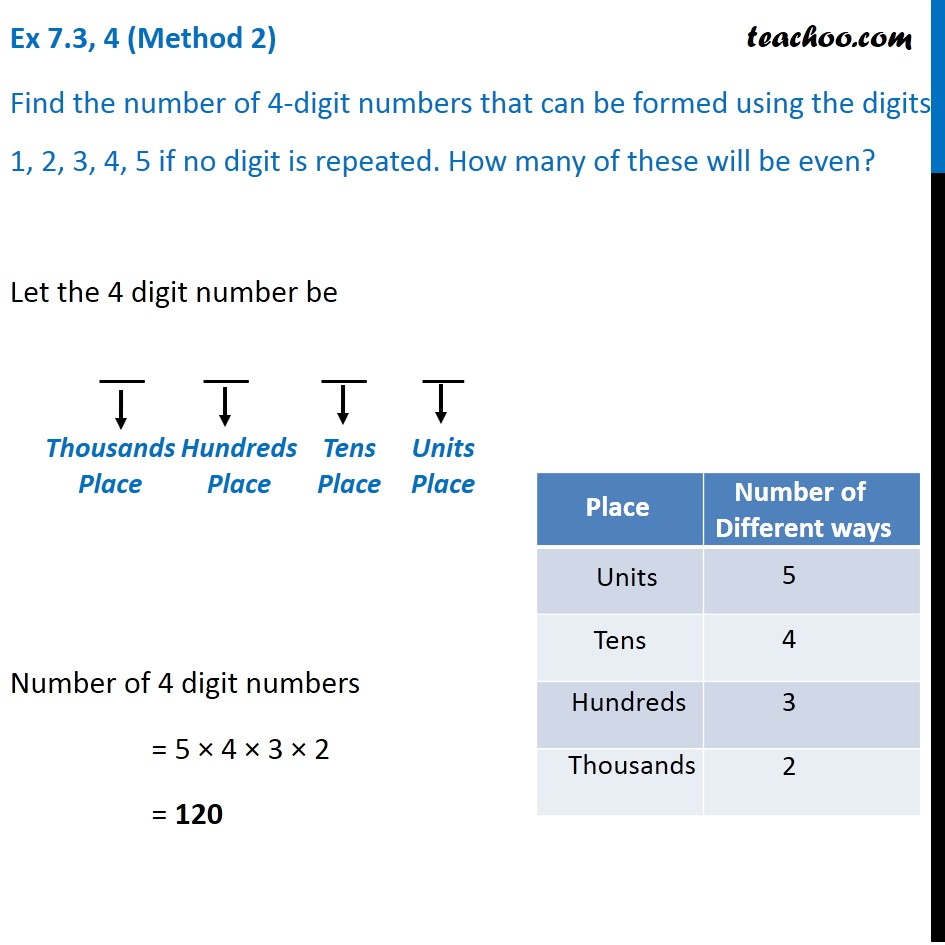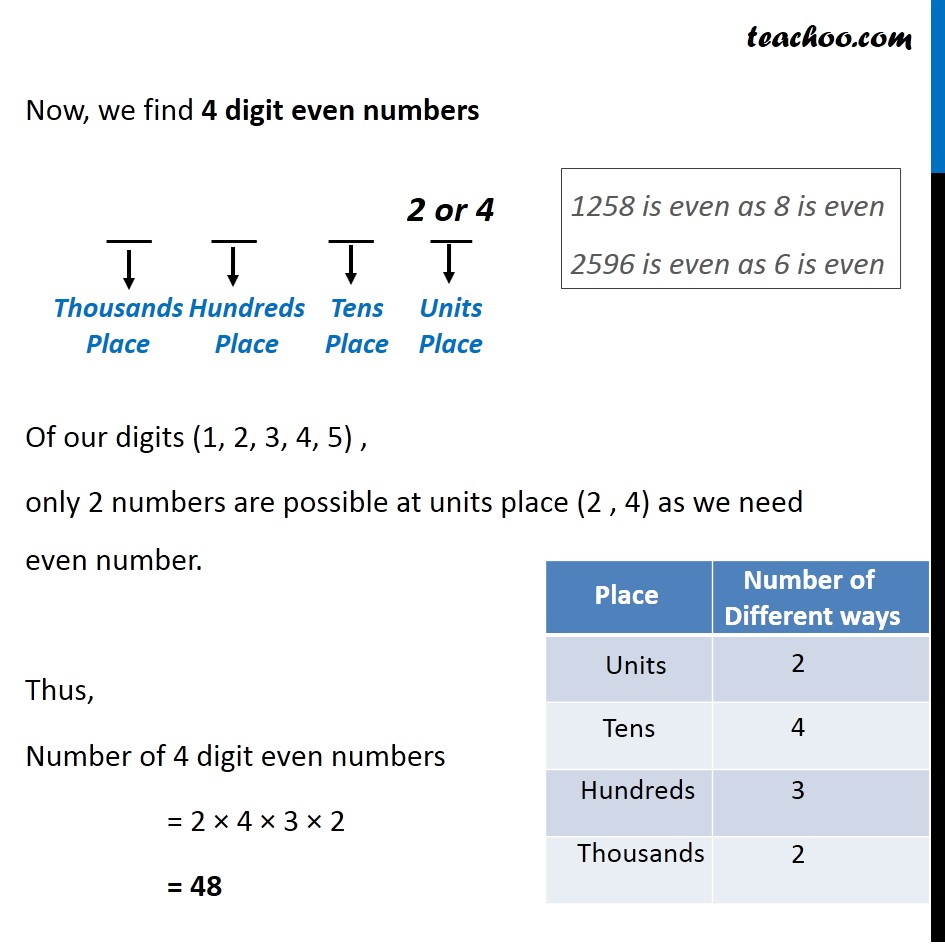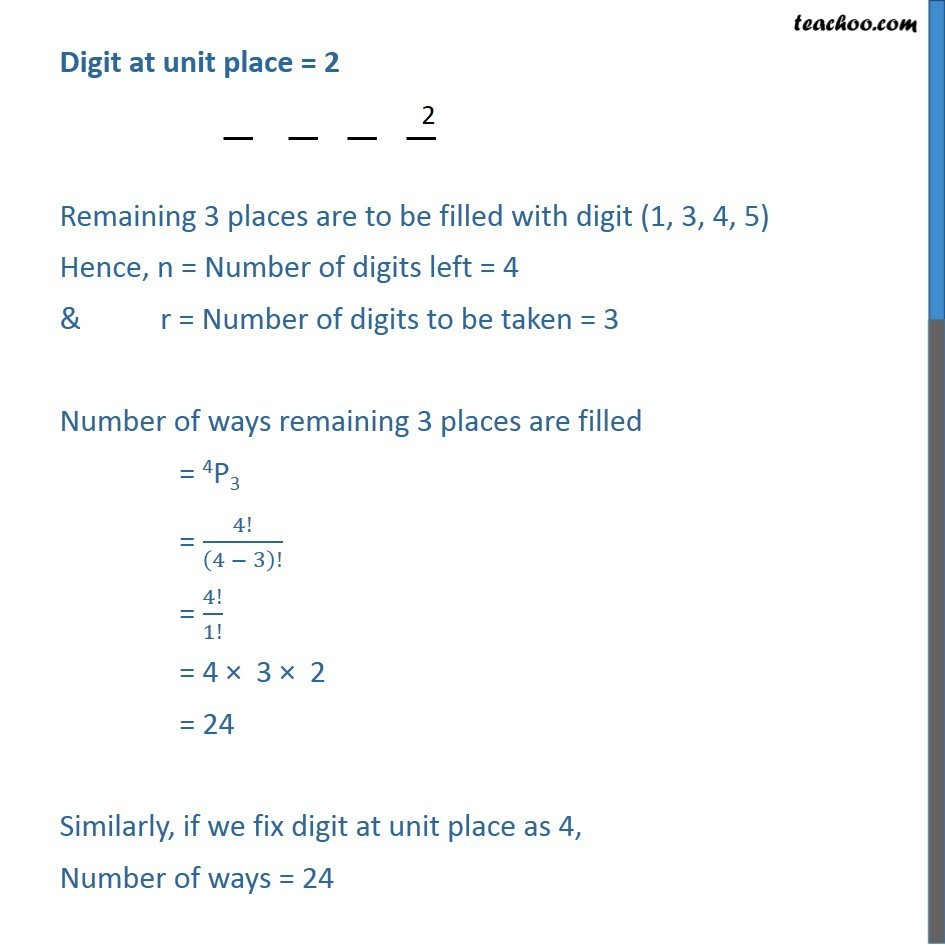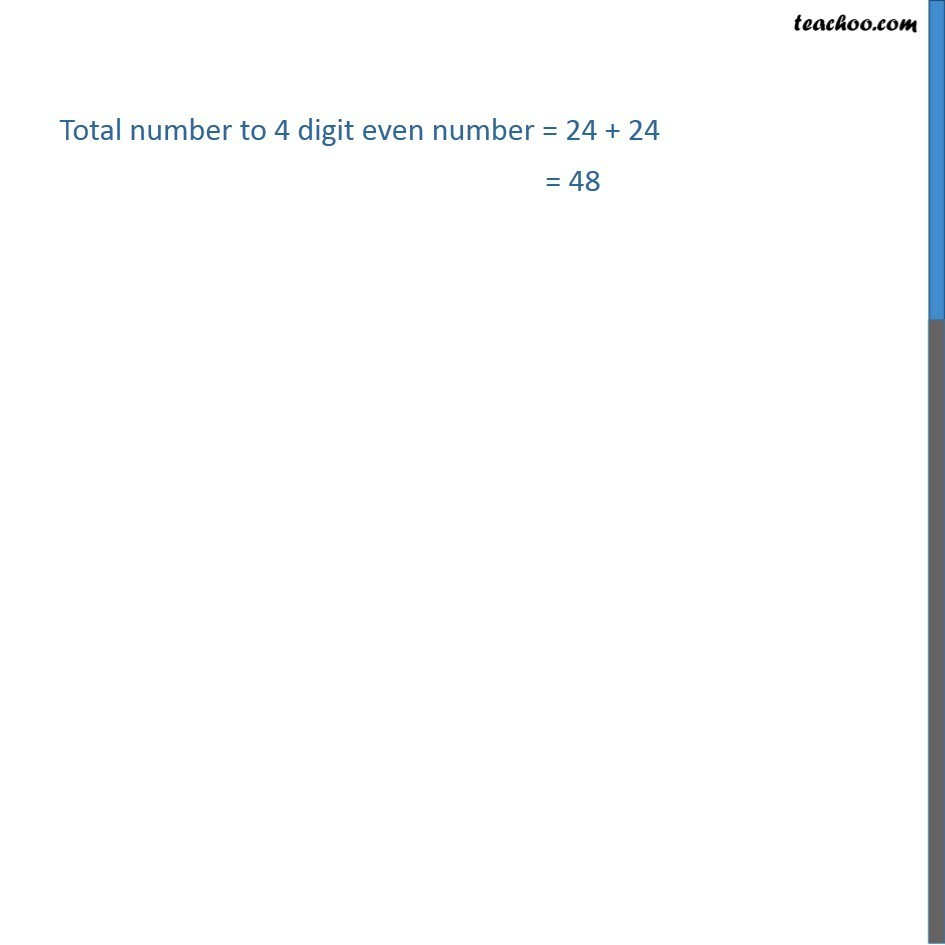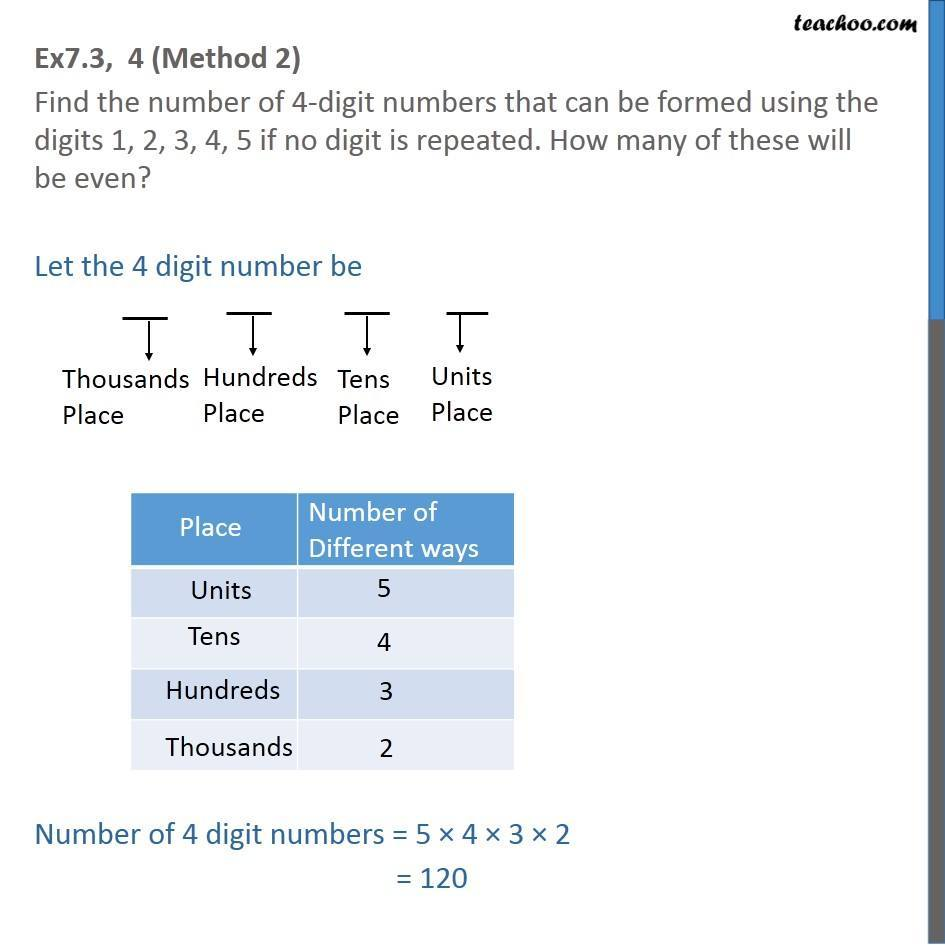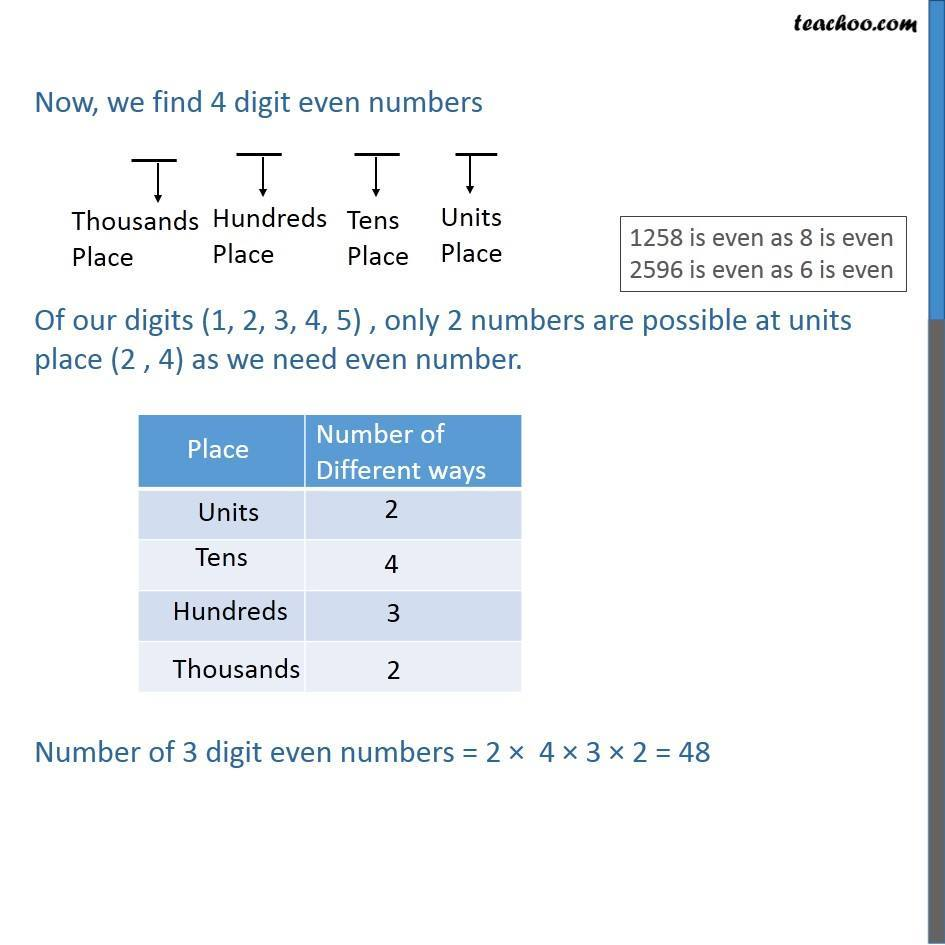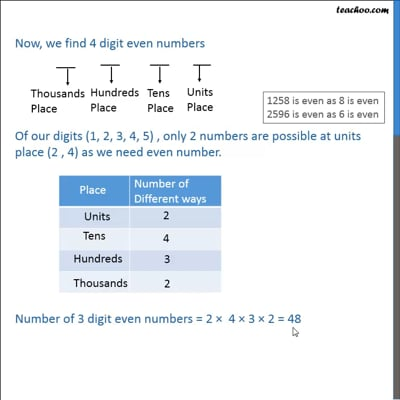This video is only available for Teachoo black users

### Transcript

Ex 7.3, 4 (Method 1) Find the number of 4-digit numbers that can be formed using the digits 1, 2, 3, 4, 5 if no digit is repeated. How many of these will be even? Let the 4 digit number be Total number of digits (1, 2, 3, 4, 5) = 5 So, n = 5 We need to make 4-digit number, so we take 4 digits at a time, So, r = 4 Number of 4-digit numbers = nPr = 5P4 = 5!/(5 − 4)! = 5!/1! = 5! = 5 × 4 × 3 × 2 × 1 = 120 Thus, number of 4-digit numbers = 120 Now, we have to find even 4-digit numbers In an even number, last digit should be even So, here units place should be even 1258 is even as 8 is even 2596 is even as 6 is even In our digits (1, 2, 3, 4, 5), only 2,4 are even Hence, either 2 or 4 can be at units place So, we fix 2 at units place and then find the total ways Numbers where Digit at unit place = 2 Remaining 3 places are to be filled with digits (1, 3, 4, 5) Hence, n = Number of digits left = 4 & r = Number of digits to be taken = 3 Number of ways remaining 3 places are filled = 4P3 = 4!/(4 − 3)! = 4!/1! = 4 × 3 × 2 = 24 Similarly, if we fix digit at unit place as 4, Number of ways = 24 Total number to 4 digit even number = 24 + 24 = 48 Ex 7.3, 4 (Method 2) Find the number of 4-digit numbers that can be formed using the digits 1, 2, 3, 4, 5 if no digit is repeated. How many of these will be even? Let the 4 digit number be Number of 4 digit numbers = 5 × 4 × 3 × 2 = 120 Thousands Place Now, we find 4 digit even numbers Of our digits (1, 2, 3, 4, 5) , only 2 numbers are possible at units place (2 , 4) as we need even number. Thus, Number of 4 digit even numbers = 2 × 4 × 3 × 2 = 48 1258 is even as 8 is even 2596 is even as 6 is even